# Section Modulus Calculator

Created by Luis Hoyos
Reviewed by Steven Wooding
Based on research by
Budynas RG, Sadegh AM Roark's Formulas for Stress and Strain (Table A.1) McGraw-Hill Education (2020)
Last updated: Jun 05, 2023

This tool calculates the section modulus, one of the most critical geometric properties in the design of beams subjected to bending. Additionally, it calculates the neutral axis and area moment of inertia of the most common structural profiles (if you only need the moment of inertia, check our moment of inertia calculator)

The formulas for the section modulus of a rectangle or circle are relatively easy to calculate. Still, when dealing with complex geometries like a tee, channel, or I beam, a calculator can save time and help us avoid mistakes.

In the following sections, we discuss the two types of section modulus, how to calculate section modulus from the moment of inertia, and present the section modulus formulas of a rectangle and many other common shapes.

## How to calculate section modulus from the moment of inertia

The section modulus is used by engineers to quickly predict the maximum stress a bending moment will cause on a beam. The equation for the maximum absolute value of the stress in a beam subjected to bending:

$\sigma_m = \frac{Mc}{I}$

, where:

• $\sigma_m$ — Maximum absolute value of the stress in a specific beam section;
• $M$ — Bending moment to which the beam is subjected in that section;
• $c$ — Largest distance from the neutral axis to the surface of the member; and
• $I$ — Second moment of area (also known as area moment of inertia) about the section neutral axis (also calculated by this tool).

For example, in a circle, the largest distance equals the radius, while it equals half the height in a rectangle.

As the ratio $I/c$ only depends on the geometric characteristics, we can define a new geometric property from it, called the section modulus, denoted by the $S$ letter:

$S = \frac{I}{c}$

As well as the second moment of area, this new geometric property is available in many tables and calculators, but if you want to know how to calculate section modulus from the moment of inertia, simply divide $I$ by $c$, and you'll get it.

Finally, we can relate the section modulus to the stress and moment:

$\sigma_m = \frac{M}{S}$

🙋 Does this relationship seem familiar to you? This relation is equivalent to the axial stress equation: $\sigma = \frac{F}{A}$. The bending moment is analogous to axial force, while the section modulus is analog to the cross-sectional area. You can use our stress calculator to obtain the axial stress.

Take these considerations into account when calculating section modulus and maximum stresses:

• We obtain the bending moment through a static or structural analysis of the beam.
• To get the section modulus, we can use tables for predefined structural members, but this calculator is the best option if you're dealing with custom geometries.
• If we're considering a uniform section beam (as usual), the location of the maximum stress will be at the point of maximum bending moment. If that's not the case, $\sigma_m$ could be at a different place.
• The previous formulas apply to materials that exhibit an elastic behavior and obey Hook's law. When there's plastic deformation instead of elastic deformation, we need to use the plastic section modulus.

## Plastic section modulus: beyond elastic section modulus

The previous equations don't apply when we subject a beam material to stresses beyond the yield strength, as they assume stress and strain are linearly related. In this case, we must use the plastic section modulus. Similar to the elastic section modulus $S$, its plastic counterpart provides a relationship between stress and moment:

$M_p = Z\sigma_Y$

, where:

• $M_p$ — Plastic moment of the section;
• $Z$ — Plastic section modulus; and
• $\sigma_Y$ — Yield strength of the member material.

The plastic moment refers to the moment required to cause plastic deformation across the whole transverse area of a section of the member.

The usefulness of the last equation is that we can predict the bending moment that will cause plastic deformation by just knowing the yield strength and plastic section modulus.

The following graphic better describes what we refer to when talking about plastic moments: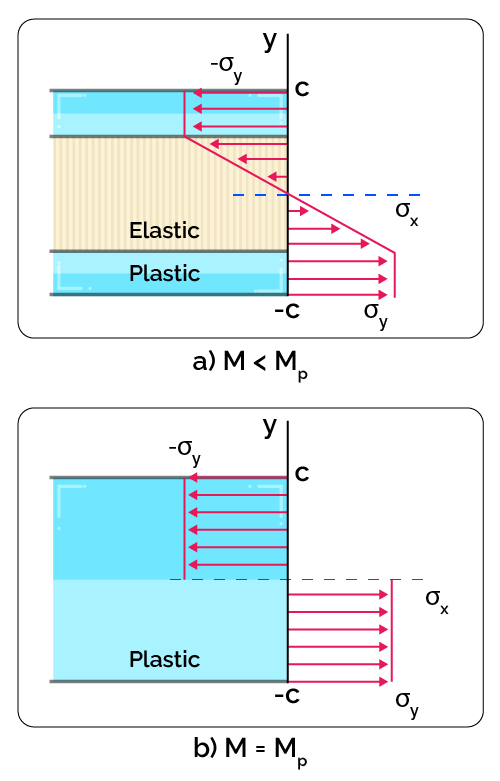Graphic representation of the bending stress distribution during plastic deformations. We're assuming a perfect plasticity model; therefore, actual stress distributions are not that uniform.

For plastic deformation to occur, we must cause some stress equal to the yield strength of the material. As you can note, the transition from elastic to plastic is not uniform across the member, as some regions will reach the yield strength before others.

Once all the section reaches the yield strength, plastic deformation occurs across all that section. The bending moment needed to achieve this is called the plastic moment.

## Section modulus formulas for a rectangular section and other shapes

In the following table, we list the section modulus formula for a rectangular section and many other profiles (scroll the table sideways to see all the equations):

Form

Elastic section Modulus

Plastic section modulus

Square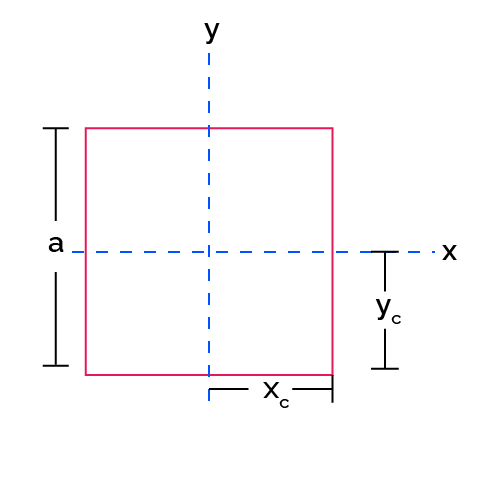$y_c = x_c = a/2$

$Z_x = Z_y = 0.25a^3$

$I_x = I_y = \frac{a^4}{12}$

$S_x = S_y =\frac{I_x}{y_c} = \frac{a^3}{6}$

Rectangle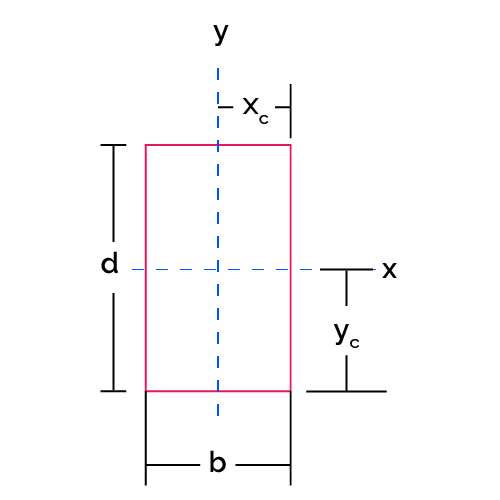$y_c=d/2$

$Z_x = 0.25bd^2$

$x_c=b/2$

$Z_y = 0.25db^2$

$I_x = \frac{bd^3}{12}$

$I_y = \frac{db^3}{12}$

$S_x = \frac{I_x}{y_c} = \frac{b d^2}{6}$

$S_y = \frac{I_y}{x_c} = \frac{d b^2}{6}$

Hollow rectangle (rectangular tube)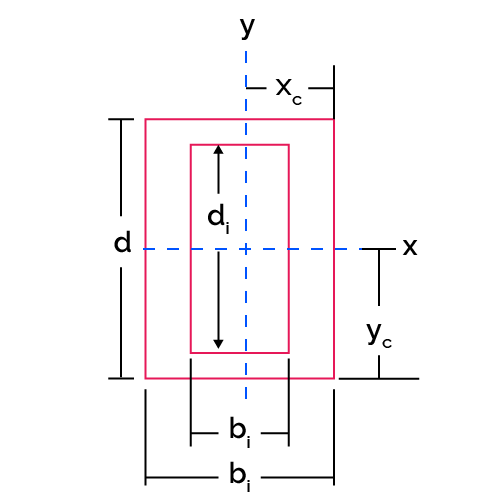$y_c=d/2$

$Z_x = 0.25(bd^2-b_id_i^2)$

$x_c=b/2$

$Z_y = 0.25(db^2-d_ib_i^2)$

$I_x = \frac{bd^3-b_id_i^3}{12}$

$I_y = \frac{db^3-d_ib_i^3}{12}$

$S_x = \frac{I_x}{y_c}$

$S_y = \frac{I_y}{x_c}$

Tee section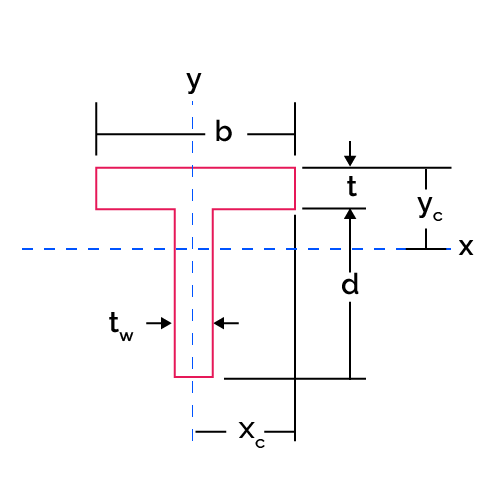$y_c=\frac{bt^2+t_wd(2t+d)}{2(tb+t_wd)}$

If $t_wd \ge bt$, then

$x_c=b/2$

$Z_x=\frac{d^2t_w}{4}-\frac{b^2t^2}{4t_w}-\frac{bt(d+t)}{2}$